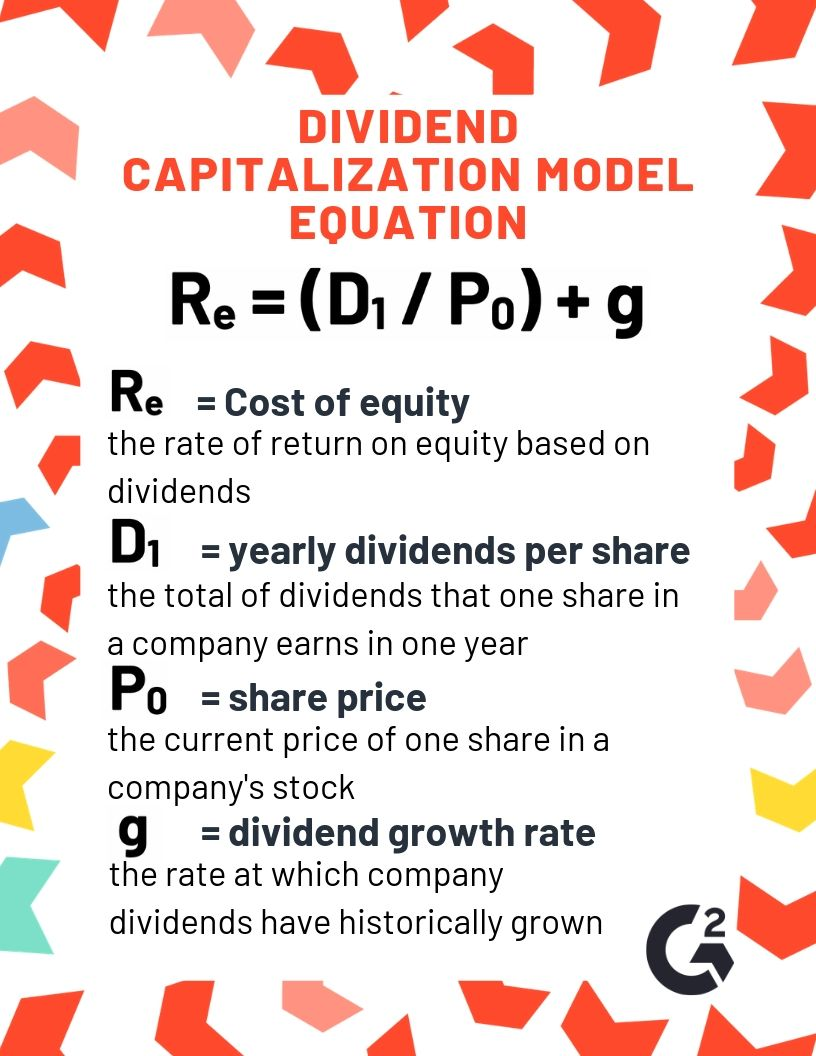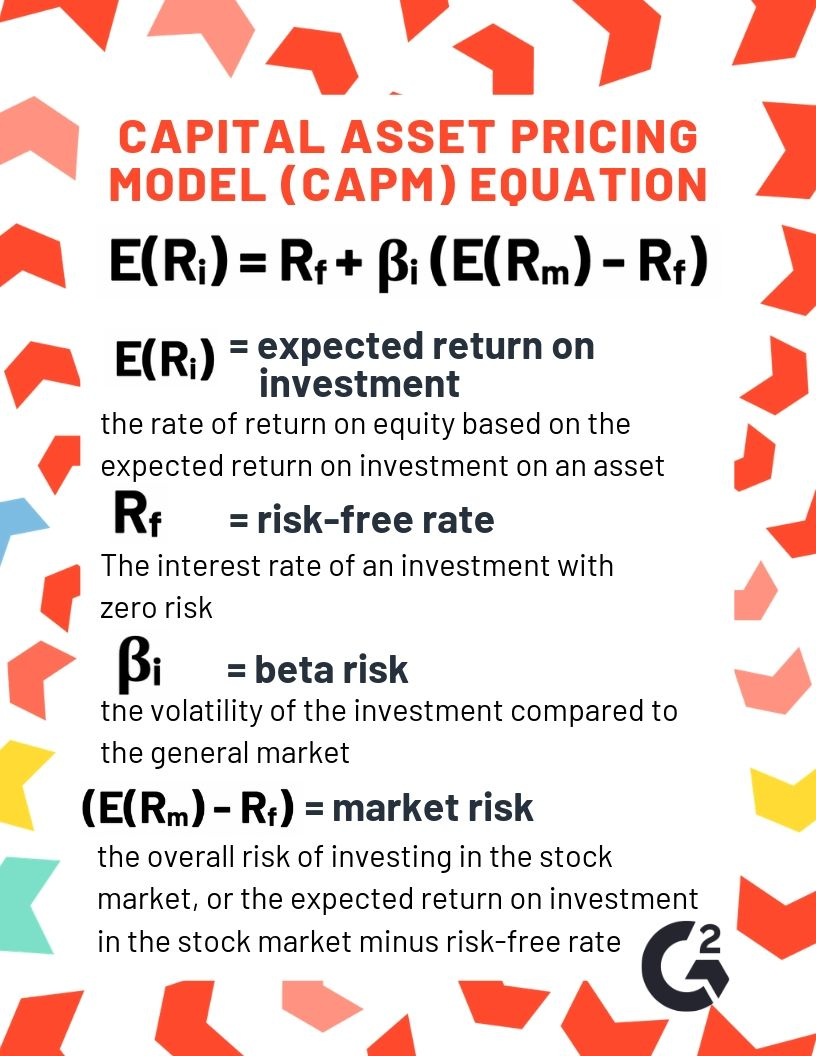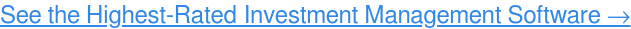If you could have any superpower, what would it be?

Stock market investors would probably choose the power to see into the future. Since they can’t exactly do that, their best bet is using equations to make predictions determining the cost of equity of their investments. Read on to learn how to predict the future for yourself using the cost of equity calculations.

# What is cost of equity?

Cost of equity is the return that an investor requires for investing in a company, or the required rate of return that a company must receive on an investment or project. It answers the question of whether investing in equity is worth the risk. It is also used, along with cost of debt, as part of the calculation of a company’s weighted average cost of capital, or WACC.

There are two ways to calculate cost of equity: using the dividend capitalization model or the capital asset pricing model (CAPM). Neither method is completely accurate because the return on investment is a calculation based on predictions about the stock market, but they can both help you make educated investments.

Take note of the formulas below:

Don’t be afraid if the symbols seem complicated—we’ll break down everything that goes into these calculations in this article.

## How do you calculate cost of equity?

The method you choose to calculate cost of equity depends on the type of investment you are analyzing and the level of accuracy you need. Remember, neither method is completely accurate (we can’t predict the future like we can when we calculate cost of debt), but both are still helpful estimates.

The dividend capitalization model requires that the stock you are analyzing earns dividends. If the investment in question does not earn dividends, you must use the CAPM formula, which is based on estimates about the company and stock market. Read on to learn what information goes into each.

### Dividend capitalization model

You already know that this model is used to determine the cost of equity of dividend stocks, so you can surmise that dividends play a part in using this equation. But what other information do you need?

Using this model, find the cost of equity of a dividend stock by dividing yearly dividends per share by the current price of one share, then adding the dividend growth rate.Keep in mind that this model does not account for stock appreciation or risk. It also presumes that the dividend payment will go up rather than stay the same or go down.

### Capital asset pricing model (CAPM)

While the CAPM for finding cost of equity is a little more complicated, it is a little bit more accurate because it accounts for the risk associated with the particular stock you are analyzing.

Using this model, find the cost of equity (or expected return of investment) by adding the risk-free rate to the beta risk of the investment multiplied by the market risk premium (found by subtracting the risk-free rate from the expected return on investment). It’s easier than it sounds—see the graphic below for an explanation of these variables.Keep in mind that this model does not account for the dividends earned by a particular stock.

## Cost of equity example

Let’s say you want to invest in a dividend stock—McDonalds, for example. Here’s how you would find the cost of equity and determine whether or not to invest.

### Dividend capitalization model example

At the time of writing, one share in McDonald’s stock is worth \$205.27. Based on the dividends McDonald’s stock earns now, we can estimate the dividends it will earn in the future by plugging that number into the equation for cost of equity using the dividend capitalization model.

According to Nasdaq, one share of McDonald’s stock earns \$1.16 quarterly. \$1.16 x 4 = \$4.64, or the yearly dividends of the stock.

The quarterly dividends for each stock last year were \$1.01. Find the dividend growth rate of the stock by dividing the current dividend payment by last year’s dividend payment, then subtracting 1. (\$1.16 / \$1.01) - 1 = 0.15 (or 15%), the dividend growth rate over one year.

 Tip: for a more accurate calculation, use the average of several years of dividend growth. Repeat the step above for multiple years, then average your results.

Plug those numbers into the dividend capitalization model equation from above, and you’ll find the cost of equity of McDonald’s stock:

 Re = 4.64/205.27 + 0.15 = 0.17 or 17%

The cost of equity, or rate of return of McDonald’s stock (using the dividend capitalization model) is 0.17 or 17%. Think that’s pretty good dividend earnings for your investing needs? Go ahead and buy McDonald’s stock! Still unsure? Worried about appreciation and risk factor? Read on, we’ll analyze the cost of equity of McDonald’s stock using CAPM next.

### Capital asset pricing model (CAPM) example

Using the CAPM, you can find the expected rate of return on any kind of asset, not just stock, but for the sake of comparison, let’s use the same McDonald’s stock we used in the example above. We will, however, need some more information about it before we calculate its cost of equity using this model.

Nasdaq tells us the beta of McDonald’s stock is 0.72. This number represents how risky investing in McDonald’s is compared to the general market.

 Tip: A beta less than one means an asset is less volatile relative to the market, and a beta greater than one means an asset is more volatile relative to the market. Of course, a beta of exactly one would mean the asset’s risk is the same as the market risk.

Think of the risk-free rate as the interest your money would earn if you just left it in the bank. You’re risking losing money by investing in stock, but your potential return will, barring disaster either to McDonald’s or the stock market/economy, be greater than just letting your money sit in the bank. According to U.S. Department of the Treasury, the risk-free rate at the time of writing is 2.17%.

The expected return on investment is the percentage of return you could expect, in general, from any investment in the stock market. The most accurate estimation of this return is provided by the S&P 500 index—it is an average rate of return of 500 of the largest publicly traded stocks in America, accounting for dividends as well as appreciation. As of writing, the average stock market return is 10%.

Now that we have all the information we need, let’s calculate the cost of equity of McDonald’s stock using the CAPM.

 E(Ri) = 0.0217 + 0.72 (0.1 - 0.0217) = 0.078 or 7.8%

The cost of equity, or rate of return of McDonald’s stock (using the CAPM) is 0.078 or 7.8%. That’s pretty far off from our dividend capitalization model calculation of 17%. That’s because instead of analyzing the yearly dividends and dividend growth, we analyzed beta and market risk.

It makes sense that our CAPM result is lower than the dividend capitalization model result because while McDonald’s stock earns high dividends compared to the rest of the market, it has a beta of less than one, meaning it is a low-risk (and therefore low-reward) investment.

#### Can we trust this calculation?

We can trust our results to an extent, but if you’ve been following along, you can see that our results from using each method are quite different. Evaluating your assets based on this calculation will not always be completely accurate, but can help point you in the right direction when it comes to investing.

On the other hand, you could also use investment portfolio management software to help you decide which stocks to invest in and how to manage your portfolio, or if you have a crystal ball that allows you to see into the future of the stock market, use that instead.What is Cost of Equity? Formula to calculate it Learn how to calculate the cost of equity of a stock using both the capital asset pricing model and the dividend capitalization model in this article. https://learn.g2.com/hubfs/cost-of-equity.jpg